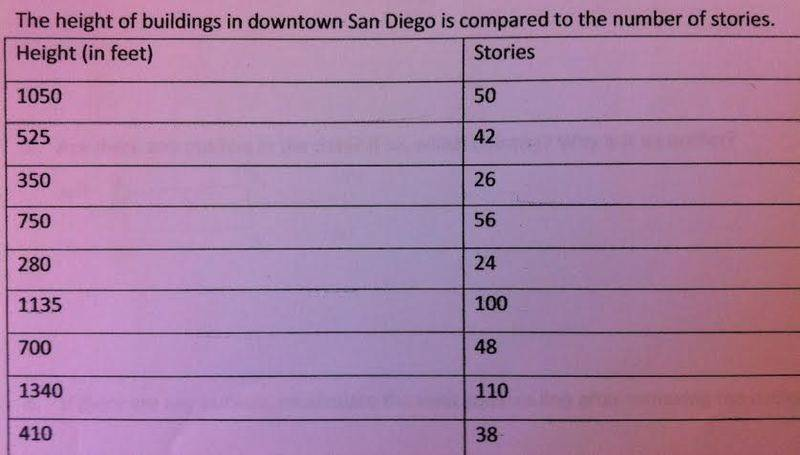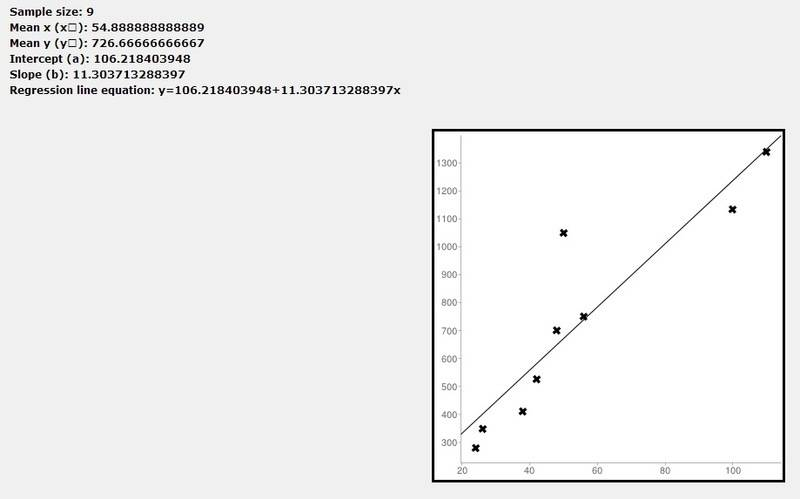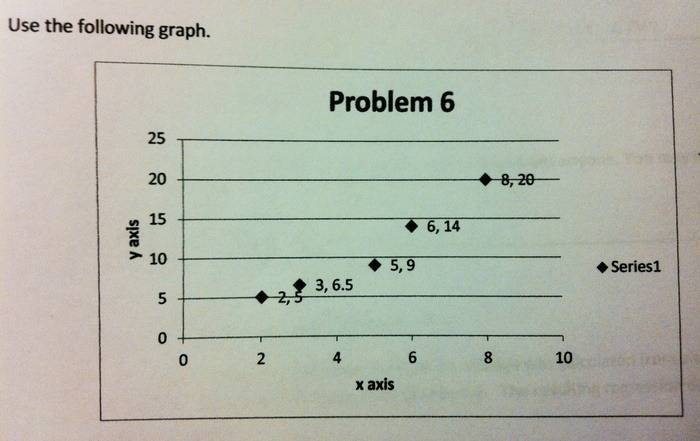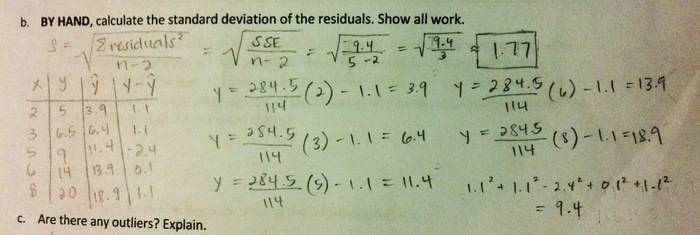# Need linear regression helpI also made a graph which is not pictured.

1.) Calculate the least squares line. Put the equation in the form of: y-hat = a + bx.
I got: y hat = 11.304 + 106.218x

a.) Find correlation coefficient. Is it significant? (use the p-value to decide)
I got: r = 0.913.... no it is not significant

b.) Are there any outliers in the data? If so which point(s)? Why is it an outlier? If there are any, recalculate the least squares line after removing the outlier(s).
--Got kinda lost here. Any help appreciated!

FactChecker
Gold Member
Plot your data and the regression line together. Is there a point that is much farther (vertically), from the line than the others? That would be an outlier. Remove that point from the data and redo the linear regression to see if it is significant without that point.

SteamKing
Staff Emeritus
Homework Helper
It's not obvious what x is supposed to represent in the regression equation. Is x supposed to be the number of stories in a building and y-hat the height of the building?

It's not obvious what x is supposed to represent in the regression equation. Is x supposed to be the number of stories in a building and y-hat the height of the building?

Yes, sorry-- "stories" is the independent variable (x) and "height" is the dependent variable (y).

SteamKing
Staff Emeritus
Homework Helper
Yes, sorry-- "stories" is the independent variable (x) and "height" is the dependent variable (y).
Then you've got a problem with your regression equation. According to it, a 10-story building would be over 1000 feet high.

Ohhh, I think I just need to switch them around, making the regression equation:

y hat = 106.218 + 11.304x

Correct?

SteamKing
Staff Emeritus
Homework Helper
Ohhh, I think I just need to switch them around, making the regression equation:

y hat = 106.218 + 11.304x

Correct?
So now, a one-story building is over 100 feet high.

Nope, that's not going to do it. I think you did something fundamentally wrong in calculating a and b.

You did use x as the number of stories and y as the heights from your data table? Look carefully, because these data are listed in reverse order in the table.

I'm confused... I also entered it into a linear regression calculator and it gave me the same equation that I got.SteamKing
Staff Emeritus
Homework Helper
This calculator is giving you the wrong results. It can calculate the mean x and mean y and add up the number of data points, but the rest is incorrect.

You can make your own calculation using a spreadsheet, and some calculators have linear regression fits built in.

SteamKing
Staff Emeritus
Homework Helper
Ohhh, I think I just need to switch them around, making the regression equation:

y hat = 106.218 + 11.304x

Correct?
I had some errors in my check calculations for a and b. This equation is correct (as is the calculator).

Sorry for the confusion.

Ok, great! It was driving me crazy-- what a relief.Moving on... I had another question for a different problem. By hand, calculate the standard deviation of the residuals.For the least square line I got:
y = 284.5/114x - 1.1
I know the residuals equation is e = y - y hat.... but I'm not exactly sure where to start?

Actually, I think I'm supposed to use the formula: SEE = √s/(n-p)

SteamKing
Staff Emeritus
Homework Helper
Ok, great! It was driving me crazy-- what a relief.Moving on... I had another question for a different problem. By hand, calculate the standard deviation of the residuals.

For the least square line I got:
y = 284.5/114x - 1.1

I know the residuals equation is e = y - y hat.... but I'm not exactly sure where to start?

For the x value of each data point, calculate y-hat according to the regression formula. The actual y value is taken from the graph. Then calculate the sum of the squares of the residuals. Divide this sum by (n-2). This is the variance. The standard deviation is the square root of the variance of the residuals.

Ok, so after calculating all of that I got: s = 15.7

Correct?

SteamKing
Staff Emeritus
Homework Helper
Ok, so after calculating all of that I got: s = 15.7

Correct?
Nope. This is way too big for s.

I accidentally squared all the y hat variables instead.. Oops. Now I have s = 3.1

SteamKing
Staff Emeritus
Homework Helper
I accidentally squared all the y hat variables instead.. Oops. Now I have s = 3.1

You're supposed to calculate the ∑ e2 and divide that by (n-2) and take the square root. Your s is still too big. Show your calculations, please.

FactChecker
Gold Member
I'm confused... I also entered it into a linear regression calculator and it gave me the same equation that I got.You can see immediately from your plot that one point at about stories = 50, height = 1050 is an outlier. That is the first data point in your table. Did you try removing that and doing the regression again? I think it will help the significance of your regression a lot.

You're supposed to calculate the ∑ e2 and divide that by (n-2) and take the square root. Your s is still too big. Show your calculations, please.
You can see immediately from your plot that one point at about stories = 50, height = 1050 is an outlier. That is the first data point in your table. Did you try removing that and doing the regression again? I think it will help the significance of your regression a lot.
Yes, I took out that outlier and got a new regression line of y hat = 43.19 + 11.59x

•FactChecker
You're supposed to calculate the ∑ e2 and divide that by (n-2) and take the square root. Your s is still too big. Show your calculations, please.SteamKing
Staff Emeritus
Homework Helper
Check your work for x = 3.

Ahh, my bad. Ok, so after fixing that, I got a new standard deviation of 1.65.

SteamKing
Staff Emeritus
Homework Helper
Ahh, my bad. Ok, so after fixing that, I got a new standard deviation of 1.65.

Looks good.

In the future, it helps if you post only one problem or question per thread. That keeps everyone from getting answers to different questions mixed up.

Noted! Sorry about that, got too excited.. But I do have one last question regarding the 1st problem I asked about... I got
Looks good.

In the future, it helps if you post only one problem or question per thread. That keeps everyone from getting answers to different questions mixed up.
Noted! Sorry about that, got too excited. But I do have one last question regarding the first problem I posted. I was to find the correlation coefficient and tell if it was significant. I got r = 0.913, and said it was not significant. Was that part correct also?

SteamKing
Staff Emeritus